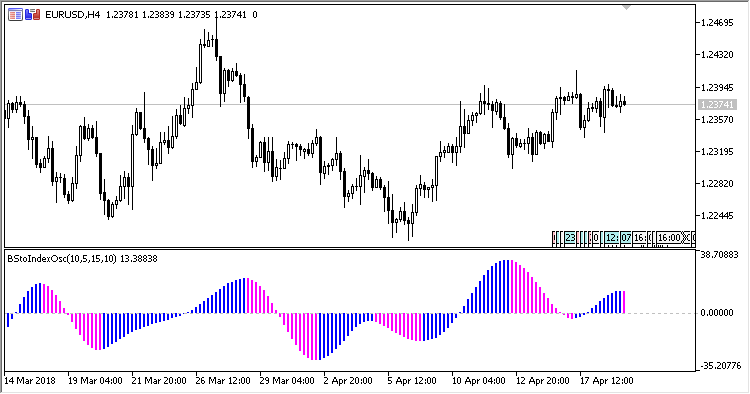# Blau_Stochastic_Index – indicator for MetaTrader 5

The Blau Stochastic Index Oscillator indicator shows the William Blau stochastic index.

It has five input parameters:

• Period – calculation period;
• First smooth period – the initial smoothing period;
• Second smooth period – the secondary smoothing period;
• Signal period – signal period;
• Method – calculation method.

Calculations:

```Stochastic[i] = 100*Diff[i]/Range[i]-50,
Signal[i] = 100*Diff_MA3[i]/Range_MA3[i]-50
```

where:

```Diff_MA3 = MA(Diff_MA2, Method, Signal period),
Diff_MA2 = MA(Diff_MA1, Method, Second period),
Diff_MA1 = MA(Diff, Method, First period),
Range_MA3 = MA(Range_MA2, Method, Signal period),
Range_MA2 = MA(Range_MA1, Method, Second period),
Range_MA1 = MA(Range, Method, First period),
Diff[i] = Close[i]-Min,
Range[i] = Max-Min,
Max, Min are the highest and the lowest price within the range from (i-Period+1) to (i)
```